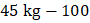# In a class there are 50 students. Their average weight is 45 kg. When a student leaves the class, the average is reduced by 100 g. Find the weight of the students who left the class? (a) 49.9 kg                               (b) 48.9 kg                          (c) 49.8 kg                   (d) 49.7 kg

## Question ID - 51410 :- In a class there are 50 students. Their average weight is 45 kg. When a student leaves the class, the average is reduced by 100 g. Find the weight of the students who left the class? (a) 49.9 kg                               (b) 48.9 kg                          (c) 49.8 kg                   (d) 49.7 kg

3537

Total weight of 50 students = 45kg.

Average weight of 49 students =g = 44.9 kg.

Total weight of 49 students =kg.Weight of the student who left the class =kg.

Next Question :
 Two balls at same temperature collide. What is conserved a) Temperature b) Velocity c) Kinetic energy d) Momentum